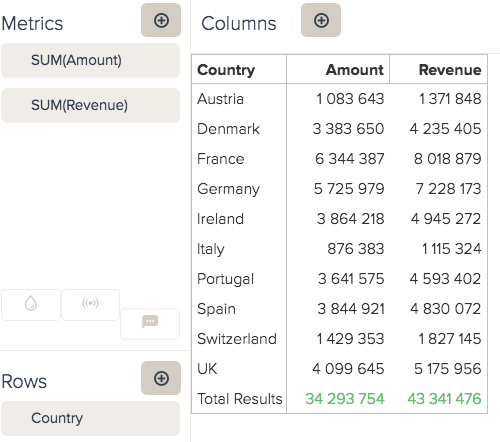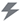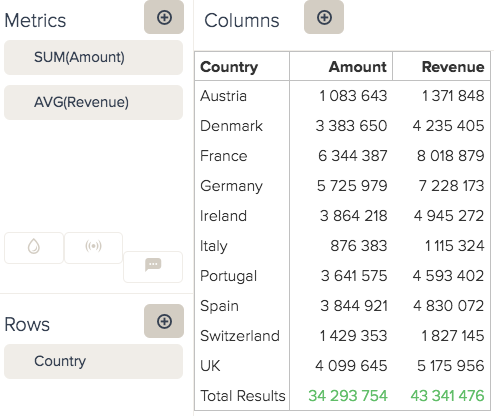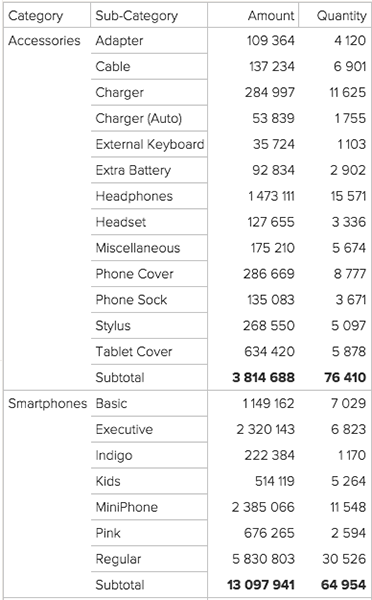Follow

In BIME, you can obtain standard sub and/or grand totals. Totals will display correctly on metrics from the original dataset. If your calculated metrics involve non-additive aggregators (AVG, COUNT, DCOUNT), they might not be computed correctly.1. In the Result manipulation menu (), select Totals.
2. Select the placement for grand and subtotals. Your selection should be based on the location of the attributes you want to add totals for.3. If you want to calculate results for non-additive totals, check the Visual totals box. By checking Visual totals, you can see the sum of the displayed values. Keep in mind that these visual totals do not match the specified aggregator. For example, if you are using the D_COUNT, the visual total will be the total of the individual results shown.4. If you want a full breakdown of the totals, check Calculate all subtotals. If you are not using Calculate all subtotals, and have multiple attributes, subtotals will be presented in groups, based on the attribute order.5. You can also select Inverse total position to position your totals row or column as the first result.
6. Click Apply. The totals filter will automatically be added to the Filters bar.
Note: When using complex calculations, some totals and subtotals can't be computed.